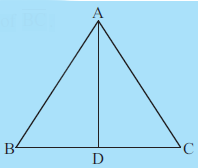Q

# In Fig 7.15, AB = AC and D is the mid - point of overline BC.

2.  In Fig 7.15,  and D is the mid-point of  .

(i) State the three pairs of equal parts in  and
(ii) Is  Give reasons.
(iii) Is  Why?Views

Here in   and

i) Three pair of equal parts are:

BD = CD ( as d is the mid point of BC)

AB = AC (given in the question)

ii) Now,

by SSS Congruency rule,

iii) As both triangles are congruent to each other we can compare them and say

.

Exams
Articles
Questions